The FREQ Procedure

### Cochran-Mantel-Haenszel Statistics

For n-way crosstabulation tables, consider the following example:

   proc freq;
tables A*B*C*D / cmh;
run;

The CMH option in the TABLES statement gives a stratified statistical analysis of the relationship between C and D, after controlling for A and B. The stratified analysis provides a way to adjust for the possible confounding effects of A and B without being forced to estimate parameters for them. The analysis produces Cochran-Mantel-Haenszel statistics, and for 2 ×2 tables, it includes estimation of the common odds ratio, common relative risks, and the Breslow-Day test for homogeneity of the odds ratios.

Let the number of strata be denoted by q, indexing the strata by h = 1, 2, ... , q. Each stratum contains a contingency table with X representing the row variable and Y representing the column variable. For table h, denote the cell frequency in row i and column j by nhij, with corresponding row and column marginal totals denoted by nhi. and nh.j, and the overall stratum total by nh.

Because the formulas for the Cochran-Mantel-Haenszel statistics are more easily defined in terms of matrices, the following notation is used. Vectors are presumed to be column vectors unless they are transposed (').

 nhi' = (nhi1,nhi2, ... ,nhiC) (1 ×C) nh' = (nh1',nh2', ... , nhR') (1 ×RC) phi · = [(nhi ·)/(nh)] (1 ×1) ph ·j = [(nh ·j)/(nh)] (1 ×1) Ph* ·' = (ph1 ·,ph2 ·, ... ,phR ·) (1 ×R) Ph ·*' = (ph ·1,ph ·2, ... ,ph ·C) (1 ×C)

Assume that the strata are independent and that the marginal totals of each stratum are fixed. The null hypothesis, H0, is that there is no association between X and Y in any of the strata. The corresponding model is the multiple hypergeometric; this implies that, under H0, the expected value and covariance matrix of the frequencies are, respectively,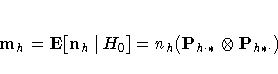and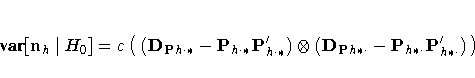where
c = [(nh2)/(nh-1)]
and where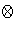denotes Kronecker product multiplication and Da is a diagonal matrix with elements of a on the main diagonal.

The generalized CMH statistic (Landis, Heyman, and Koch 1978) is defined as

Q CMH = G'VG-1G
where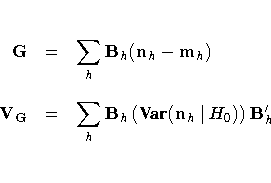and where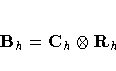is a matrix of fixed constants based on column scores Ch and row scores Rh. When the null hypothesis is true, the CMH statistic has an asymptotic chi-square distribution with degrees of freedom equal to the rank of Bh. If VG is found to be singular, PROC FREQ prints a message and sets the value of the CMH statistic to missing.

PROC FREQ computes three CMH statistics using this formula for the generalized CMH statistic, with different row and column score definitions for each statistic. The CMH statistics that PROC FREQ computes are the correlation statistic, the ANOVA (row mean scores) statistic, and the general association statistic. These statistics test the null hypothesis of no association against different alternative hypotheses. The following sections describe the computation of these CMH statistics.

Caution: The CMH statistics have low power for detecting an association in which the patterns of association for some of the strata are in the opposite direction of the patterns displayed by other strata. Thus, a nonsignificant CMH statistic suggests either that there is no association or that no pattern of association has enough strength or consistency to dominate any other pattern.

#### Correlation Statistic

The correlation statistic, popularized by Mantel and Haenszel (1959) and Mantel (1963), has one degree of freedom and is known as the Mantel-Haenszel statistic.

The alternative hypothesis for the correlation statistic is that there is a linear association between X and Y in at least one stratum. If either X or Y does not lie on an ordinal (or interval) scale, then this statistic is not meaningful.

To compute the correlation statistic, PROC FREQ uses the formula for the generalized CMH statistic with the row and column scores determined by the SCORES= option in the TABLES statement. See the section "Scores" for more information on the available score types. The matrix of row scores Rh has dimension 1 ×R, and the matrix of column scores Ch has dimension 1 ×C.

When there is only one stratum, this CMH statistic reduces to (n-1)r2, where r is the Pearson correlation coefficient between X and Y. When nonparametric (RANK or RIDIT) scores are specified, then the statistic reduces to (n-1)rs2, where rs is the Spearman rank correlation coefficient between X and Y. When there is more than one stratum, then this CMH statistic becomes a stratum-adjusted correlation statistic.

#### ANOVA (Row Mean Scores) Statistic

The ANOVA statistic can be used only when the column variable Y lies on an ordinal (or interval) scale so that the mean score of Y is meaningful. For the ANOVA statistic, the mean score is computed for each row of the table, and the alternative hypothesis is that, for at least one stratum, the mean scores of the R rows are unequal. In other words, the statistic is sensitive to location differences among the R distributions of Y.

The matrix of column scores Ch has dimension 1 ×C, the column scores are determined by the SCORES= option.

The matrix of row scores Rh has dimension (R-1) ×R and is created internally by PROC FREQ as

Rh = [ IR-1 , -JR-1 ]
where IR-1 is an identity matrix of rank R-1, and JR-1 is an (R-1) ×1 vector of ones. This matrix has the effect of forming R-1 independent contrasts of the R mean scores.

When there is only one stratum, this CMH statistic is essentially an analysis of variance (ANOVA) statistic in the sense that it is a function of the variance ratio F statistic that would be obtained from a one-way ANOVA on the dependent variable Y. If nonparametric scores are specified in this case, then the ANOVA statistic is a Kruskal-Wallis test.

If there is more than one stratum, then this CMH statistic corresponds to a stratum-adjusted ANOVA or Kruskal-Wallis test. In the special case where there is one subject per row and one subject per column in the contingency table of each stratum, this CMH statistic is identical to Friedman's chi-square. See Example 28.8 for an illustration.

#### General Association Statistic

The alternative hypothesis for the general association statistic is that, for at least one stratum, there is some kind of association between X and Y. This statistic is always interpretable because it does not require an ordinal scale for either X or Y.

For the general association statistic, the matrix Rh is the same as the one used for the ANOVA statistic. The matrix Ch is defined similarly as

Ch = [ IC-1 , -JC-1 ]
PROC FREQ generates both score matrices internally. When there is only one stratum, then the general association CMH statistic reduces to QP (n-1)/n, where QP is the Pearson chi-square statistic. When there is more than one stratum, then the CMH statistic becomes a stratum-adjusted Pearson chi-square statistic. Note that a similar adjustment can be made by summing the Pearson chi-squares across the strata. However, the latter statistic requires a large sample size in each stratum to support the resulting chi-square distribution with q(R-1)(C-1) degrees of freedom. The CMH statistic requires only a large overall sample size since it has only (R-1)(C-1) degrees of freedom.

Refer to Cochran (1954); Mantel and Haenszel (1959); Mantel (1963); Birch (1965); Landis, Heyman, and Koch (1978).

#### Adjusted Odds Ratio and Relative Risk Estimates

The CMH option provides adjusted odds ratio and relative risk estimates for stratified 2 ×2 tables. For each of these measures, PROC FREQ computes the Mantel-Haenszel estimate and the logit estimate. These estimates apply to n-way table requests in the TABLES statement, when the row and column variables both have only two levels.

For example,

   proc freq;
tables A*B*C*D / cmh;
run;

In this example, if the row and columns variables C and D both have two levels, PROC FREQ provides odds ratio and relative risk estimates, adjusting for the confounding variables A and B.

The choice of an appropriate measure depends on the study design. For case-control (retrospective) studies, the odds ratio is appropriate. For cohort (prospective) or cross-sectional studies, the relative risk is appropriate. See the section "Odds Ratio and Relative Risks for 2×2 Tables" for more information on these measures.

Throughout this section, z denotes the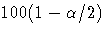percentile of the standard normal distribution.

Odds Ratio, Case-Control Studies

Mantel-Haenszel Estimator The Mantel-Haenszel estimate of the common odds ratio is computed as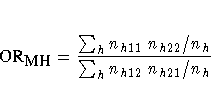It is always computed unless the denominator is zero. Refer to Mantel and Haenszel (1959) and Agresti (1990).

Using the estimated variance for log( OR MH) given by Robins, Breslow, and Greenland (1986), PROC FREQ computes the corresponding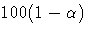% confidence limits for the odds ratio as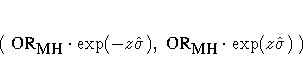where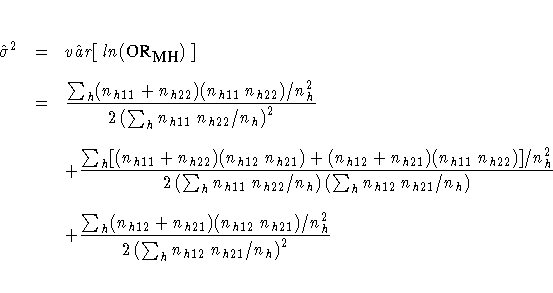Note that the Mantel-Haenszel odds ratio estimator is less sensitive to small nh than the logit estimator.

Logit Estimator The adjusted logit estimate of the odds ratio (Woolf 1955) is computed as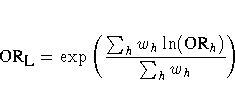and the corresponding% confidence limits are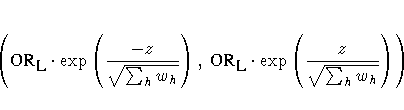where ORh is the odds ratio for stratum h, and
wh = [1/(var(ln ORh))]

If any cell frequency in a stratum h is zero, then PROC FREQ adds 0.5 to each cell of the stratum before computing ORh and wh (Haldane 1955), and prints a warning.

Relative Risks, Cohort Studies

Mantel-Haenszel Estimator The Mantel-Haenszel estimate of the common relative risk for column 1 is computed as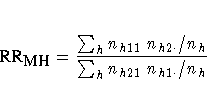It is always computed unless the denominator is zero. Refer to Mantel and Haenszel (1959) and Agresti (1990).

Using the estimated variance for log( RR MH) given by Greenland and Robins (1985), PROC FREQ computes the corresponding% confidence limits for the relative risk as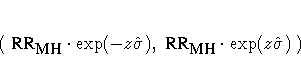where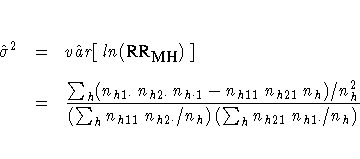Logit Estimator The adjusted logit estimate of the common relative risk for column 1 is computed as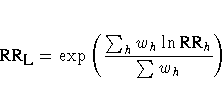and the corresponding% confidence limits are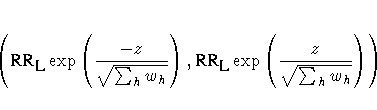where RRh is the column 1 relative risk estimate for stratum h, and
wh = [1/(var (ln RRh))]
If nh11 or nh21 is zero, then PROC FREQ adds 0.5 to each cell of the stratum before computing RRh and wh, and prints a warning. Refer to Kleinbaum, Kupper, and Morgenstern (1982, Sections 17.4 and 17.5).

#### Breslow-Day Test for Homogeneity of the Odds Ratios

When you specify the CMH option, PROC FREQ computes the Breslow-Day test for stratified analysis of 2 ×2 tables. It tests the null hypothesis that the odds ratios for the q strata are all equal. When the null hypothesis is true, the statistic has an asymptotic chi-square distribution with q-1 degrees of freedom.

The Breslow-Day statistic is computed as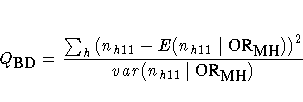where E and var denote expected value and variance, respectively. The summation does not include any table with a zero row or column. If OR MH equals zero or if it is undefined, then PROC FREQ does not compute the statistic and prints a warning message.

Caution: Unlike the Cochran-Mantel-Haenszel statistics, the Breslow-Day test requires a large sample size within each stratum, and this limits its usefulness. In addition, the validity of the CMH tests does not depend on any assumption of homogeneity of the odds ratios; therefore, the Breslow-Day test should never be used as such an indicator of validity.

Refer to Breslow and Day (1994).